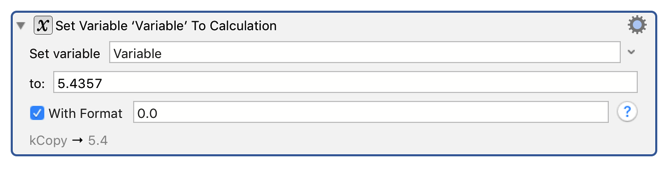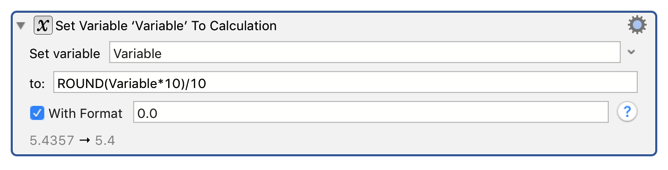# Round to 1 decimal place

Been trying to find how to convert say 5.4357 to 5.4. Any ideas how?

Set Variable MyVar to Calculation MyVar with format 0.0.Or more explicitly with ROUND(Variable*10)/10:3 Likes

Oh I didn’t know that Format thing was there! So when you set the Format, does it still store the actual number in the variable to more decimal places? Or does it strip those off for calculations too?

I’m finding I am only getting integers. Must be doing something wrong. Here is my calculation:

``````Time left: %Calculate%ROUND((CurrentTime-TimeStart)*FileCount/LoopCount/60*10)/10% minutes
``````

Anything obvious I am doing wrong? I want one decimal place.

Edit: The code above does work. I edited a duplicate window. Doh!

Variables are strings, so what you see is what they are, there are no hidden digits.

The calculation looks fine. Note that in that calculation, if the number happens to end in .0, then the .0 will not show up, because the Calculate token does not have any formatting.

Since then, the CalculateFormat token was added, which would allow you to explicitly format it with one decimal place:

``````Time left: %CalculateFormat%ROUND((CurrentTime-TimeStart)*FileCount/LoopCount/60*10)/10%0.0% minutes
``````
1 Like